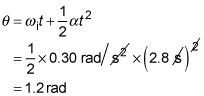##### Physics I: 501 Practice Problems For Dummies (+ Free Online Practice)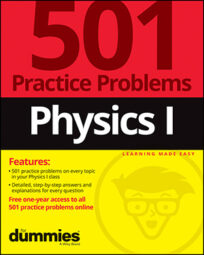Using physics, you can calculate the angular acceleration of an object in circular motion. For example, you can find the angular acceleration of a car's front passenger-side tire as the car accelerates.

Here are three problems for you to practice finding angular acceleration.

## Practice questions

1. When you switch your room fan from medium to high speed, the blades accelerate at 1.2 radians per second squared for 1.5 seconds. If the initial angular speed of the fan blades is 3.0 radians per second, what is the final angular speed of the fan blades in radians per second?

2. The radius of a car tire is about 0.35 meters. If the car accelerates in a straight line from rest at 2.8 meters per second squared, what is the angular acceleration, both magnitude and direction, of the front passenger-side tire?

3. A merry-go-round has an angular acceleration of 0.30 radians per second squared. After accelerating from rest for 2.8 seconds, through what angle in radians does the merry-go-round rotate?

The following are the answers to the practice questions:

Angular acceleration is defined by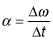where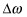is the final angular speed minus the initial angular speed and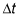is the time over which the angular speed changes. You know the initial angular speed of the fan blades, so you can write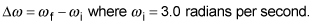Solve the equation for acceleration for the final angular speed and plug in the known quantities to get the answer. The result is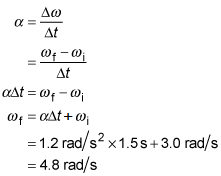The angular acceleration is related to the linear acceleration by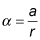In this case, a = 2.8 meters per second squared and r = 0.35 meters.

Plug these quantities into the equation: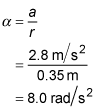By using the right-hand rule, you find that the direction of the angular acceleration is to the left of the car when facing the direction in which the car moves.

The acceleration of the merry-go-round is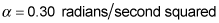and it accelerates for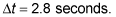Its initial angular speed is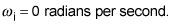Plug these values into the equation for angular displacement to get